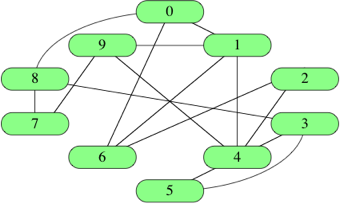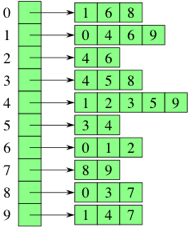# Graph representation

Graphs are connections between entities.

• The social network is a graph.

## Glossary

term definition
acyclic graph a path with no cycles
adjacent or incident when two vertices are connected
cycle a path that reconnects with the origin vertex
dag an acyclic graph
degree the amount of edges between two vertices
edge a line representing the connection between two vertices
directed graph a graph which edges have one direction flow
in-degree the number of edges pointing into a vertex
out-degree the number of edges pointing out of a vertex
neighbors a vertex refering to another adjacent vertex
path the sequential edges that connect two vertices
shortest path the path with less edges
sparse graph graph with relatively few edges
to leave when a directed edge points out of a vertex
to enter when a directed edge points into a vertex
undirected graph a graph which edges go both ways
vertex a single entity
vertices entities in the graph

## Weighted graph

The edges can have a numeric weight.

For example, a weight can be the distance between two cities (vertices).

## Use cases

• Social networks
• flowcharts
• dependency flow charts

## Representation• vertices are mostly names as numbers.
• |V| means all vertices from 0 to V - 1

### Criteria

1. How much memory or space we need in each representation
2. How long will it take to determine whether a given edge is in the graph
3. How long it takes to find the neighbors of a given vertex

### Types

#### 1. Edge list

An edge list is an array of |E| edges.

• an edge can be an object or a sub-array
• you can add the weight there
##### Example
const graph = [ [0,1], [0,6], [0,8], [1,4], [1,6], [1,9], [2,4], [2,6], [3,4], [3,5],
[3,8], [4,5], [4,9], [7,8], [7,9] ]

##### Evaluation
• A search would be more of a linear search unless optimized
• Space is Θ(|E|)

A matrix of |V| x |V|

• Symmetric matrix if it is an undirected graph• The matrix can be represented as an array of rows (sub-arrays)
• The intersections state if there is an edge between x and y
const graph =
[ [0, 1, 0, 0, 0, 0, 1, 0, 1, 0],
[1, 0, 0, 0, 1, 0, 1, 0, 0, 1],
[0, 0, 0, 0, 1, 0, 1, 0, 0, 0],
[0, 0, 0, 0, 1, 1, 0, 0, 1, 0],
[0, 1, 1, 1, 0, 1, 0, 0, 0, 1],
[0, 0, 0, 1, 1, 0, 0, 0, 0, 0],
[1, 1, 1, 0, 0, 0, 0, 0, 0, 0],
[0, 0, 0, 0, 0, 0, 0, 0, 1, 1],
[1, 0, 0, 1, 0, 0, 0, 1, 0, 0],
[0, 1, 0, 0, 1, 0, 0, 1, 0, 0] ]

##### Pros
• Search in constant time eg. graph
##### Cons
• Takes Θ($V^2$) space
• To determine which vertices are adjacent to a vertex x it takes a linear search through all the x row

Fusion of Edge lists and Adjacency matrices

• On each row, store only the adjacent vertices to that vertex.const graph = [
[1, 6, 8],    // 0
[0, 4, 6, 9], // 1
[4, 6],       // etc...
[4, 5, 8],
[1, 2, 3, 5, 9],
[3, 4],
[0, 1, 2],
[8, 9],
[0, 3, 7],
[1, 4, 7]
];

##### Pros
• Can get into any vertex in constant time.
• We can find the neighbors in constant time.
##### Space
• It takes 2 x |V| or Θ(|V + E|) since each vertex is recorded twice in the graph.